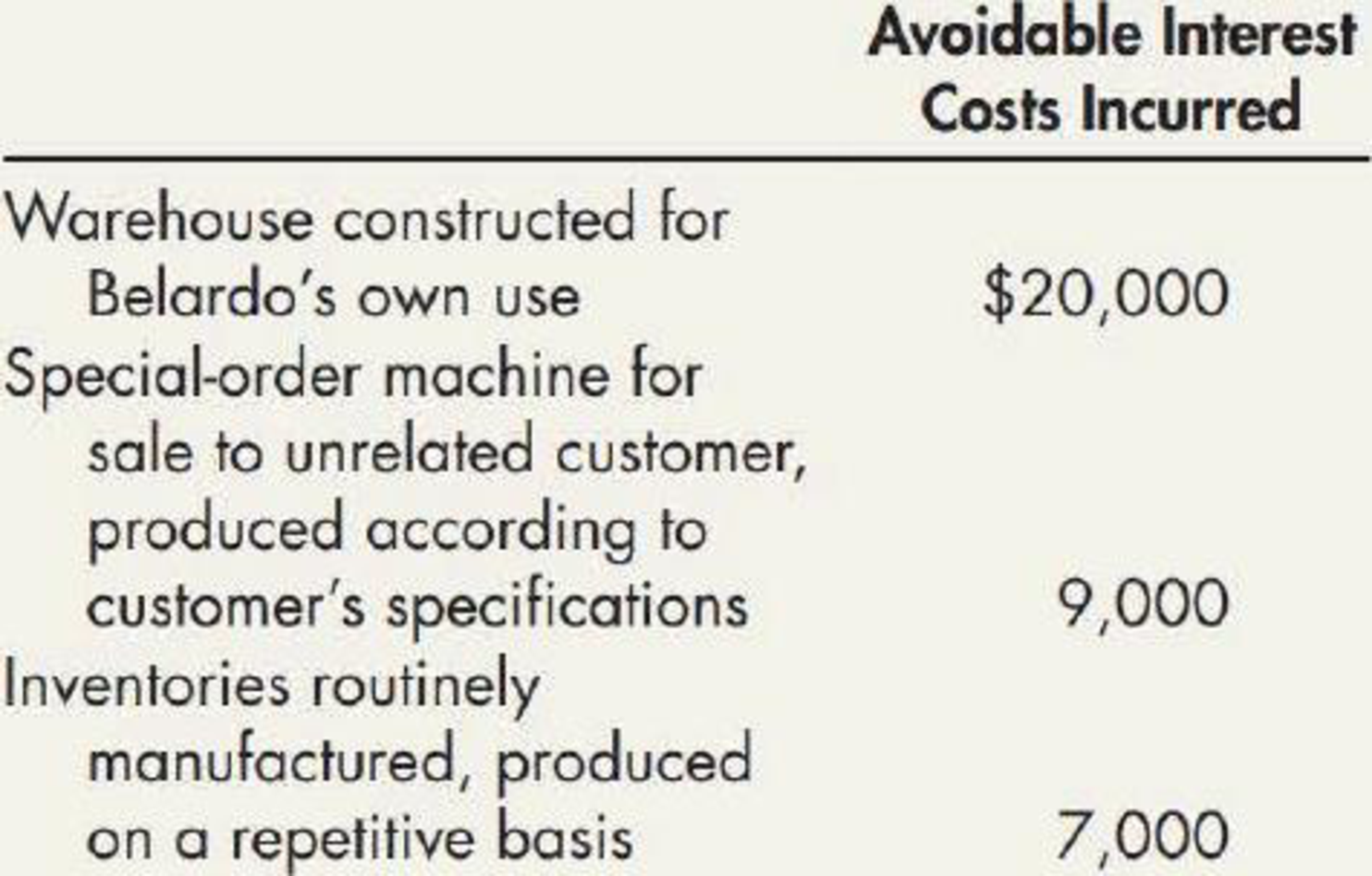Chapter 10, Problem 7MC### Intermediate Accounting: Reporting...

3rd Edition
James M. Wahlen + 2 others
ISBN: 9781337788281

#### Solutions

Chapter
Section### Intermediate Accounting: Reporting...

3rd Edition
James M. Wahlen + 2 others
ISBN: 9781337788281
Textbook Problem
309 views

# Belardo Corporation constructed and manufactured certain assets and incurred the following avoidable interest costs in connection with those activities:All of these assets required an extended period of time for completion. Assuming that the effect of interest capitalization is material, what is the total amount of interest costs to be capitalized? a. $0 b.$20,000 c. $29,000 d.$36,000

To determine

Calculate the amount of total interest to be capitalized.

Explanation

Capitalized interest:

Interest Cost incurred to finance the construction of a long-term construction projects are known as capitalized interest.

Justification for the incorrect option a, b and d:

$0,$20,000 and \$36,000 are not the amount of interest to be capitalized.

Justification for the correct option c:

Calculate the amount of interest to be capitalized:

Amountofinteresttobecapitalized}=(WarehouseconstructedforCorpor

### Still sussing out bartleby?

Check out a sample textbook solution.

See a sample solution

#### The Solution to Your Study Problems

Bartleby provides explanations to thousands of textbook problems written by our experts, many with advanced degrees!

Get Started

#### Find more solutions based on key concepts